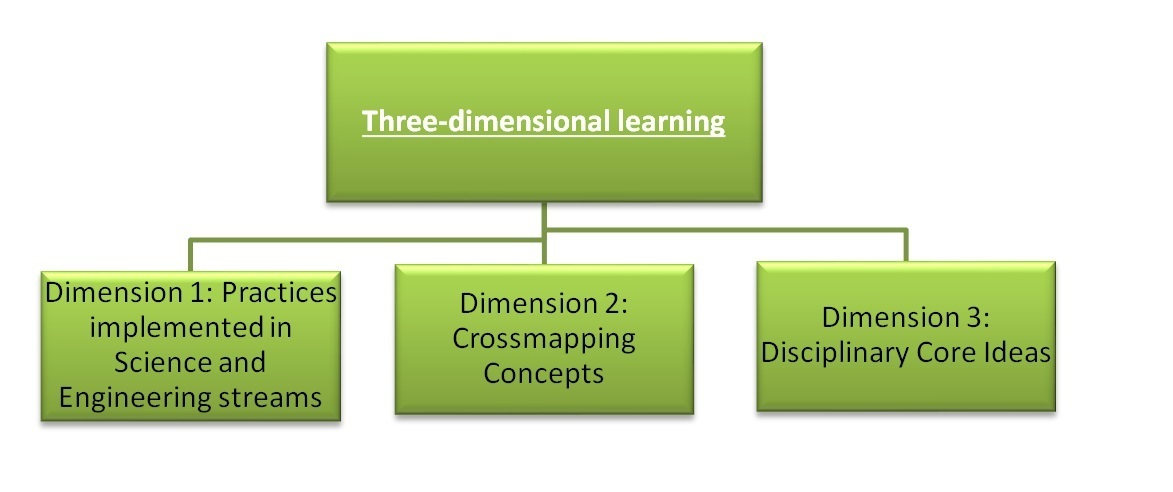## Why 3D Learning Is The Future Of Education

In order to understand various complex theories in mathematics, science, and engineering and their application in real-life scenarios, it becomes essential to encourage students to visualize, evaluate, and analyze a solution for a problem from all angles. This means that students are encouraged to find the solution to a complex problem by looking at it from different angles. By using this approach, the students will start thinking out-of-the-box, and embark on a journey of constantly discovering new knowledge and developing their skills with ease.

### The Concept Of 3D Learning

It was very important that the students understood all concepts thoroughly; for this, K-12 curriculum development companies introduced the concept of 3D learning in eLearning courseware as per the National Research Council's (NRC) framework. The core idea behind introducing three-dimensional learning by K-12 content providers was to render high-quality science education, strengthen students' science knowledge, and boost their performance on exams. It does so by focusing on:

• Dimension 1: Practices implemented in science and engineering streams
• Dimension 2: Cross-mapping concepts
• Dimension 3: Disciplinary core ideas### Dimension 1: Practices Implemented In Science And Engineering Streams

The first dimension involves asking students to see a problem through the eyes of scientists (working in the science and engineering sectors) in order to solve different and complex real-world problems. Most of the K-12 curriculum development companies use about eight science and engineering practices as per the guidelines provided by the National Research Council. These practices are:

• Inculcating the habit of probing problems and defining solutions
• Asking questions (investigation), considering a problem from all possible angles
• Evaluating and construing the available data
• Implementing mathematics and a systematic computational approach
• Developing  different solution models
• Cross-questioning solution models from the available evidence
• Communicating information to gather in-depth details
• Coming out with explanations and solutions with a deep understanding of the respective matter

### Dimension 2: Cross-Mapping Concepts

Cross-mapping concepts involve:

• Identifying the different patterns of problems
• Understanding the underlying source and effects
• Preparing models
• Establishing a connection between patterns and models in different scientific disciplines

The main agenda behind introducing cross-mapping concepts by K-12 content providers was to foster students' habits of applying concepts learned in one scientific discipline to another. For example, students can understand the properties and behavior of gases in physics and apply it to earth science, pharmacy, chemistry, biology, engineering, and other science disciplines.

### Dimension 3: Disciplinary Core Ideas

Disciplinary core ideas are geared toward strengthening knowledge related to science-based theories and concepts. The focus on disciplinary core ideas helped K-12 curriculum development companies give equal, far-reaching importance across various science and engineering disciplines.

For example, 3D animation helped students comprehend a concept related to polyhedron on X, Y, and Z planes (3D view). Similarly, word problems based on volume, work and time, trigonometry, and calculus became easy to visualize and understand with 3D animation. Similarly, questions and answers, when explained in three dimensions, were easier to follow. To make the eLearning solution more learner-centric, K-12 content development companies in India introduced assessments, group projects, and tests along with 2D and 3D animations to evaluate students' understanding.

K-12 content providers create K–12 science curriculum, instruction, and assessments so that students utilize the taught scientific or technological knowledge in real life and use science concepts as key tools in investigating, comprehending, and solving various complex scientific scenarios. K-12 curriculum development companies designed and structured K-12 courseware in such a way that it was simple and learnable across different grades.

Close